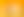Sampling The Olympics

# Stages1. An Introduction to Sampling

EXPLORE

Suggested Learning Intentions

• To understand that sampling is necessary to make inferences about a population
• To be able to explain and use at least two different sampling techniques

Sample Success Criteria

• I can explain how a sample could be used to make inferences about a population
• I can use a recognised sampling technique to select a sample
• I can model and justify my thinking using a range of manipulatives
EXPLORE

2. Podiums and the Pool

EXPLORE

Suggested Learning Intentions

• To use different tools to represent information, to solve a problem or support an opinion
• To understand the concepts of ‘and’ and ‘or’ when used in the context of probability

Sample Success Criteria

• I can construct a two-way table and a Venn diagram to represent information
• I can use these graphical displays to help solve problems
• I can use a range of manipulatives to model and justify my solutions
• I can explain the meaning of 'and' and 'or' as used in probability
EXPLORE

3. Olympic Attitudes

EXPLORE

Suggested Learning Intentions

• To understand that different samples taken from the same population can provide different statistical measures, and lead to different inferences being made about the population
• To recognise that bias can influence the sampling process

Sample Success Criteria

• I can explain how the sampling process can be influenced by bias
• I can compare statistics drawn from different samples of the same population
• I can explain why statistics from different samples of the same population could show different information
• I can explain and justify my thinking using a range of manipulatives
EXPLORE

4. Does Hosting Help?

EXPLORE

Suggested Learning Intentions

• To synthesise and analyse data from a variety of sources to make inferences
• To informally identify outliers and explain their impact

Sample Success Criteria

• I can use data that includes outliers to make inferences about a population
• I can identify outliers and explain their impact on a data set
• I can explain my thinking and solutions using a range of manipulatives
EXPLORE